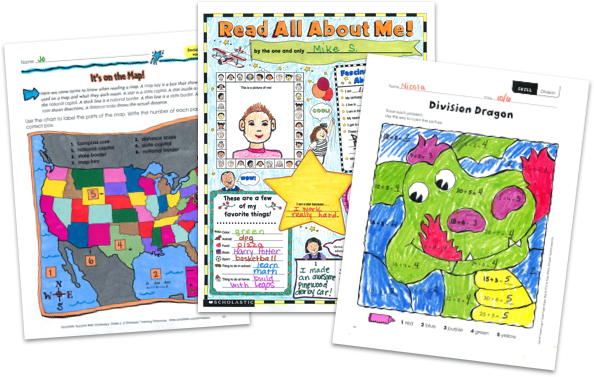MY FILE CABINET# 3rd Grade Math Worksheets From Scholastic TeachablesScholastic Teachables (formerly Scholastic Printables) has more than 1,500 third grade math worksheets that cover a variety of different math skills! Worksheets include addition and subtraction with multi-digit numbers, decimal operations, multiplication, summation and subtraction of currency, simple geometry, and more, all in easy-to-digest and engaging printables. Our worksheets are all professionally produced for 3rd graders.

Scholastic Teachables (formerly Scholastic Printables) has more than 1,500 third grade math worksheets that cover a variety of different math skills! Worksheets include addition and subtraction with multi-digit numbers, decimal operations, multiplication, summation and subtraction of currency, simple geometry, and more, all in easy-to-digest and engaging printables. Our worksheets are all professionally produced for 3rd graders.

## Scholastic's 3rd Grade Math Worksheets Cover:

• Charts and Graphs
• Decimals
• Estimation
• Fractions
• Geometry
• Money Math
• Multiplication and Division
• Patterns and Symmetry
• Perimeter and Area
• Place Value
• Probability
• Real-World Math
• Skip Counting
• Time and Measurement
• Word Problems

## Sample Third Grade Math Worksheets

More than 1,500 printable 3rd grade math worksheets and activities from Scholastic span multiple math topics to make learning fun and engaging. Here's a sample of math worksheets for you to try in your class FREE with a 30-day trial or subscription.

## Scholastic's 3rd Grade Math Worksheets Cover:

• Charts and Graphs
• Decimals
• Estimation
• Fractions
• Geometry
• Money Math
• Multiplication and Division
• Patterns and Symmetry
• Perimeter and Area
• Place Value
• Probability
• Real-World Math
• Skip Counting
• Time and Measurement
• Word Problems
• Charts and Graphs
• Decimals
• Estimation
• Fractions
• Geometry
• Money Math
• Multiplication and Division
• Patterns and Symmetry
• Perimeter and Area
• Place Value
• Probability
• Real-World Math
• Skip Counting
• Time and Measurement
• Word Problems

## Sample Third Grade Math Worksheets

More than 1,500 printable 3rd grade math worksheets and activities from Scholastic span multiple math topics to make learning fun and engaging. Here's a sample of math worksheets for you to try in your class FREE with a 30-day trial or subscription.## Additional Alphabet Worksheets (Subscription Required)

Try FREE for 30 days!

If you are not 100% satisfied cancel during the FREE trial and owe nothing. If you are not 100% satisfied cancel during the FREE trial and owe nothing.